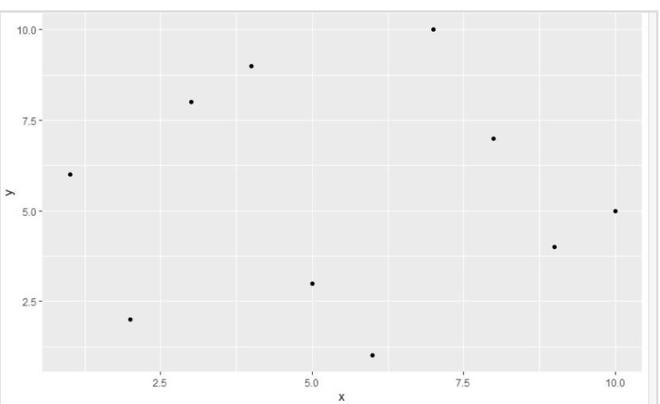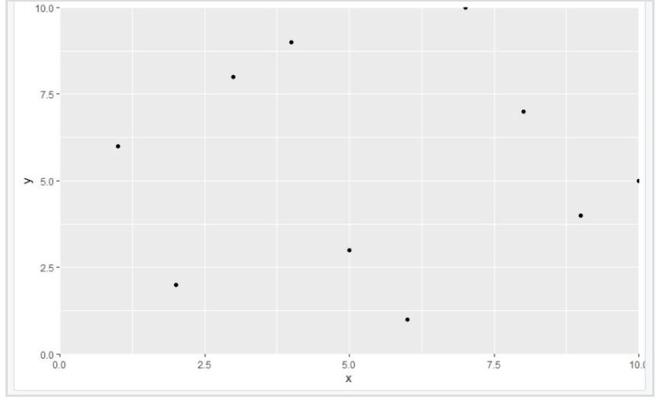# Set Origin of ggplot2 Plot Axes to Zero in R

• Last Updated : 31 Aug, 2021

In this article, we will be looking at the approach to set the origin of the gplot2 plot axes to zero with the help of some functions of R programming language.

In this approach to set the origin of the ggpot2 plot axes to zero, the user first needs to install and import the ggplot2 package in the working R console, and then call the scale_x_continuous() and scale_y_continuous() functions together with the plot, with the required parameters and this will add zeroes to the origin of both the ggplot2 plot axes in the R programming language,

Scale_x_continuous() and scale_y_continuous() functions functions are used to set the continuous position scales (x & y).

Syntax:

scale_x_continuous(…, expand = waiver())

scale_y_continuous(…, expand = waiver())

Parameters:

• …: common continuous scale parameters: name, breaks, labels, na.value, limits, and trans.
• Expand: a numeric vector of length two giving multiplicative and additive expansion constants.

Let us first look at initial plot, so that the difference is apparent.

Example: initial plot

## R

 `library``(ggplot2)`` ` `gfg < - ``data.frame``(x=``c``(4, 9, 5, 6, 10, 2, 3, 7, 8, 1),``                   ``y=``c``(9, 4, 3, 1, 5, 2, 8, 10, 7, 6))`` ` `gfg_plot < - ``ggplot``(gfg, ``aes``(x, y)) + ``geom_point``()`` ` `gfg_plot`

Output:Now, let’s add zeroes to origin. using the approach described above.

Example: final plot

## R

 `library``(ggplot2)`` ` `gfg < - ``data.frame``(x=``c``(4, 9, 5, 6, 10, 2, 3, 7, 8, 1),``                   ``y=``c``(9, 4, 3, 1, 5, 2, 8, 10, 7, 6))`` ` `gfg_plot < - ``ggplot``(gfg, ``aes``(x, y)) + ``geom_point``()+``scale_x_continuous``(expand=``c``(0, 0), limits=``c``(0, 10)) +``scale_y_continuous``(expand=``c``(0, 0), limits=``c``(0, 10))`` ` `gfg_plot`

Output:My Personal Notes arrow_drop_up